Banding in DAX - Excelerator BI

# Banding in DAX

Level: Intermediate

As regular readers of my blog would know, I enjoy helping people on forums who need help with DAX, Power Query or Power BI.  Recently I have answered quite a few questions for people that could benefit from a DAX “Banding” solution and hence I have decided to write this post as a permanent link to the topic.  I first learnt about the DAX banding technique from Alberto Ferrari at http://sqlbi.com

## What is Banding?

Banding is a technique that allows you to “group” or “stratify” your data in a table into “bands” (it is sometimes called Cohort analysis).  So let’s say you you have a table of customers like this (shown below).  In my sample database I have 18,000 customers and I know the age of each one.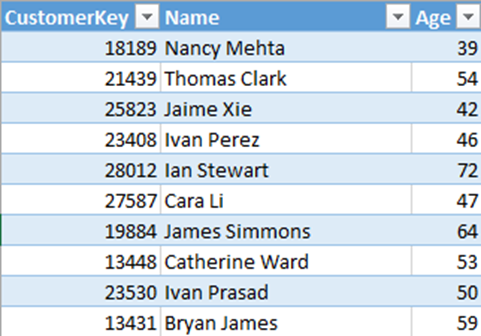This provides an opportunity to analyse the sales data based on age so as to understand if people of different ages purchase differently.  Below is a pivot table that illustrates what is possible with the data.  But the problem is that there is far too much detail to be useful.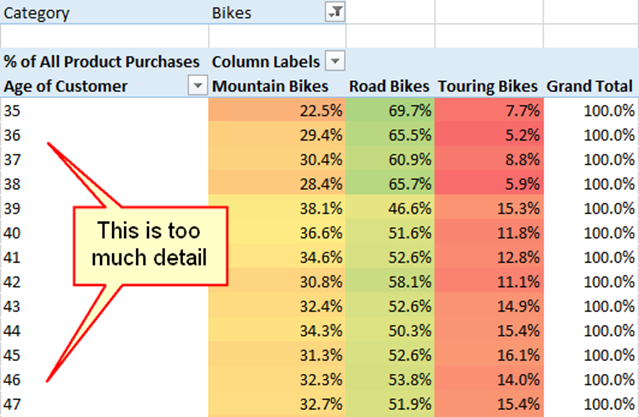I don’t really want to target 35 year old customers differently to 36 year old customers.  Ideally I would like to group (or stratify) the customers into “bands” so that I can treat a group of similar customers the same way.  So I might want to group my customers like this.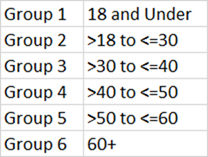Once I have grouped customers by age, I can then create a pivot table like the one shown below.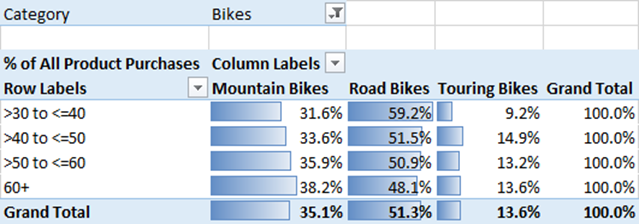Notice how much easier it is to find insights into the data when there is less detail.  I can immediately see from the above pivot table that as people get older, they tend to be more interested in mountain bikes and touring bikes and less interested in road bikes (of course this is a fake database, so the insights are not necessarily realistic).

## You will need a Calculated Column for Banding

I am a strong advocate for using Measures (not calculated columns) when ever possible.  You can read more about that in my Knowledge Base here.  But in this instance, a Measure is not going to work.  Measures can only be used in the values section of a Pivot Table – you can’t use them in the “filtering” areas like Slicers, Rows, Columns, and Filters.  In the pivot table above, the bands are used to slice the pivot table on rows, and hence a measure wont work.

## I Could Write an If Statement

So the objective is to create a calculated column in the customers table that groups people based on age into a stratified band.  Any Excel user would know that you could write an IF statement for that (DAX has very similar syntax for the IF statement in regular Excel).  The DAX syntax for a single IF statement is as follows

`= IF(Customers[Age] <= 18, "18 and Under", "Over 18")`

The DAX Calculated Column above will return one of two possible values depending on the age of the customer.  The complexity comes in when you want to have multiple conditions.  As I mentioned earlier in this post, there are 6 possible groups that I have in mind (shown here again).To do this with IF statements, I would need to nest multiple IF statements inside each other as follows.

```=
IF(
Customers[Age] <= 18,
"18 and Under",
IF(
Customers[Age] > 18
&& Customers[Age] <= 30,
">18 and <=30",
IF(
Customers[Age] > 30
&& Customers[Age] <= 40,
">30 and <=40",
IF(
Customers[Age] > 40
&& Customers[Age] <= 50,
">40 and <=50",
IF(Customers[Age] > 50 && Customers[Age] <= 60, ">50 and <=60", ">60" )
)
)
)
)```

Do you see the problem?  This Calculated Column works, but it is very hard to write, read and modify.  And more importantly, there is a better way – use the banding technique.## Process for Creating Banding

The better way involves the following process.

1. Create a table in Excel that contains the group names and the lower/upper age limits
2. Load the table to Power Pivot
3. Do not connect the table to any other tables – it is a disconnected table
4. Write a DAX Calculated Column that compares the age of each customer against the lower/upper limits in your disconnected table and returns the single row from the banding table that matches for each customer.

Here is the detail of how to do it.

### Create a Table in Excel

Here is what a table in Excel looks like.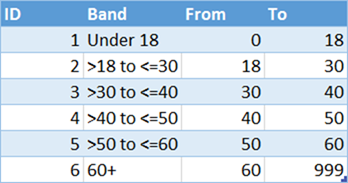The key features to note are

1. There is and ID column – this will be used to sort the Band column later
2. A “Band” column – this is the label that describes each group.  Note the groups are mutually exclusive and collectively exhaustive (MECE).
3. There is a “from” and “to” column that set the lower and upper bounds of each age group.  Note that the upper age for one group will match the lower age for the next group.  I have used DAX to ensure no overlap in the Calculated Column.

### Write a Calculated Column

The objective of the calculated column is to filter the disconnected Banding table so that 1 and only 1 row is “visible” or “unfiltered” for each customer.  Here is the formula

```= CALCULATE(
VALUES(AgeBands[Band]),
FILTER(AgeBands,
Customers[Age] > AgeBands[From] &&
Customers[Age] <= AgeBands[To]
)
)```

The role of the FILTER portion of this formula is to filter the AgeBands table so only a single row is unfiltered. What the FILTER does is apply 2 filter rules to ensure the customer is older than the “from” column and “less than or equal” to the “to” column.  It is this less than or equal to portion that ensures no cross over of individuals into multiple groups (Mutually Exclusive).

The VALUES function has a unique capability.  VALUES returns a single column table.  If that table also has a single row, then VALUES converts the table to a scalar value and allows that value to used as a result in a calculated column or indeed in a pivot table cell.  Given the formula guarantees that there is 1 and only 1 row left unfiltered in this banding table, then VALUES will kick in, extract the label for the group from the AgeBands[Band] column and place that value in the calculated column.

### Sorting the New Calculated Column

There is one more problem to solve – the names of the new groups do not sort properly in the Pivot Table.  By default, columns of data will sort in Alphanumeric order.  So the labels will sort like this: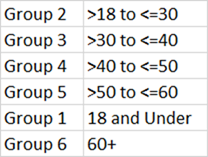To solve this problem, I created a second calculated column that brings in the Sort ID into the customer table.  It is simple to do this – just copy the formula in the first calculated column and then update the VALUES portion with the “ID” column as follows.

```= CALCULATE(
VALUES(AgeBands[ID]),
FILTER(AgeBands, Customers[Age] > AgeBands[From] && Customers[Age] <=AgeBands[To])
)```

Once this second calculated column has been created, it is possible to tell Power Pivot to use the new column of Sort ID values as the sort order for the Age Band column (shown below).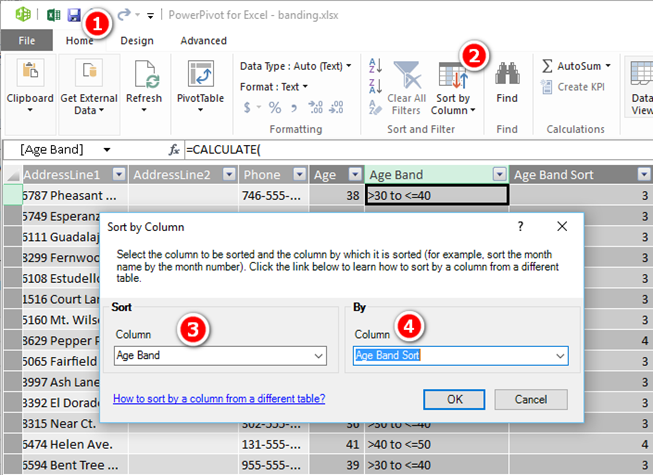### Compression of the Calculated Columns

As I mentioned earlier, I am not a big fan of calculated columns – except where they are truly needed.  There are a few guidelines about when it is OK to use a calculated column – you can read about them all in the link I provided to my Knowledge Base earlier.  The key points to note here however are.

1. These calculated columns are on the lookup table (Customers).  Calculated columns on lookup tables are generally OK.
2. The calculated columns have a low cardinality (ie a low number of unique values).  This is also generally OK, even on a large data table if necessary.

### Now to Use the New Calculated Column

Now that the Customers table has this new “Age Band” column, it is possible to use this column in visualisations.  It can be used on rows, columns, filters, and slicers.  Here is a rows example: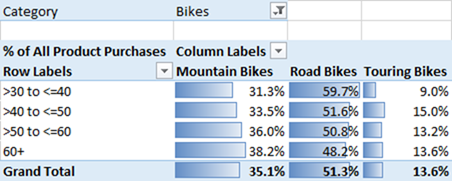And a slicer example: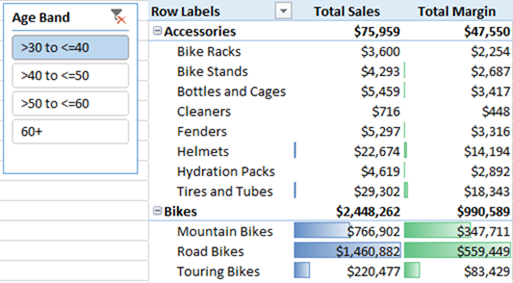## Learn to Write DAXThe banding topic is just one of the many techniques I cover in my books “Supercharge Power BI” and “Supercharge Excel“.  If you are a Power BI user or an Excel user and are learning to use DAX, my books give a comprehensive coverage of all the topics you need to start your journey as a Power Pivot ninja.

### 30 thoughts on “Banding in DAX”

1.Hi Matt,

Thank you for this tutorial. It was incredibly clear and explained everything with the right amount of detail. I was able to perform exactly what I needed to.

2.Guillermo Velazquez

Hi, thanks a lot. This is very helpful and well explained.

I’ve adapted your solution to a price-banding report, the calculated colum is pretty much the same. But I keep getting the following error:
“Calculation error in column ‘REPORT_TABLE'[]: A table of multiple values was supplied where a single value was expected”
(where “REPORT_TABLE” has the prices I want to bucket, along many other columns)

I’m not very fond of PP or DAX (I’d use SQL, but I’m limited on which tools to use). So, so far I’m able, I cannot identify what’s the problem. I can only imagine it could be the table’s design, if it is not the formula.

I’d really appreciate any help.

1.I suggest you check 2 things.
1. Make sure your age brackets in the banding table don’t overlap.
2. Make sure the > and <= logic in your calculated columns is like I have written

3.Hi Mat

I have managed to create the banding column as per your article but am still struggling to create the sort order column. My scenario is as follows:

I have a duration in minutes column on my fact table and this is at an event level, i.e. the event took x minutes to complete. My banding table is a separate table with 10 rows, the columns are a from and to column, a description and a sort column.

From To Time Band Sort Order
0 60 0 to 1 Hour 1
61 120 1 to 2 Hours 2
121 180 2 to 3 Hours 3
181 240 3 to 4 Hours 4
241 300 4 to 5 Hours 5
301 360 5 to 6 Hours 6
361 480 6 to 8 Hours 7
481 720 8 to 12 Hours 8
721 1440 12 to 24 Hours 9
1441 999999 Over 1 Day / 24 Hours 10

I have creating a calculated column on the fact using the following formula:

=CALCULATE (
VALUES (‘Time Band'[Time Band] ),
FILTER (
‘Time Band’,
[Duration Minutes] >= ‘Time Band'[From Minute]
&& [Duration Minutes] = ‘Time Band'[From Minute]
&& [Duration Minutes] <= 'Time Band'[To Minute]
)
)

And I get the following error (Events is my fact table, Time Band is the first calculated column and Calculated Column 1 is the attempted sort order column)
Failed to save modifications to the server. Error returned: 'A circular dependency was detected: Events[Calculated Column 1], Events[Time Band], Events[Calculated Column 1].
'.

The only thing I can see different from your example is that you have these columns on a dimension type table, whereas mine is on a fact table. I event tried to make the sort order column on my banding table a row identifier but it did not help.

This is driving me NUTS!

Thanks
Trevor

1.Sorry the formula for the sort calculated column did not post. Its the same as the first one except is uses the sort column (‘Time Band'[Sort Order])

2.I understand the problem, and it is complicated. It is related to the fact that there is no unique column ID on the fact table. As fate would have it, there was a great blog I read today by my friend Danill Maslyuk that shows an alternate way you can write the sort column. https://xxlbi.com/blog/sort-by-column/

1.Hi Matt. I looked at the article and although we will be using Power BI desktop to surface a dashboard to the users, the real model lies in Analysis Services Tabular as the users also do their own analysis by browsing the cube directly. For now, I am going to use the age old way of sorting of a number that is stored as text and have my bands as 00 to 01 Hour, 01 to 02 Hour…..12 to 24 Hours. I believe Noah used it on the ark when doing roll call of all the animals….

4.HI Matt

I have implemented a duration banding solution using your method described in this article. The grain of my fact table is event dates and times and the time band shows how long it takes between events. Your solution works great. Thanks. Is there a way to have this banding dynamic so that if I look at a higher level than event date and time (e.g. the group that the events belong to), then the band adjusts to show the duration of all events in the same group. Example, I have 10 events with dates and times and each event will have its own band (0 to 1 hour, 1 to 2 hours, etc.). But all 10 events took a collective 14 hours to complete and this should show 10 to 15 hours as the band if I remove the events and show the band next to the group name. Here is my existing formula
=CALCULATE (
VALUES (‘Time Band'[Time Band] ),
FILTER (
‘Time Band’,
[Duration Minutes] >= ‘Time Band'[From Minute]
&& [Duration Minutes] <= 'Time Band'[To Minute]
)
)
Hope this makes sense

5.Sorry, I’m not clear what the second formula is, or why you need 2. My example is on a lookup table and it has a primary key. It looks like yours is on a data table and my guess is that doesn’t have a primary key. This can make a difference. But adding a primary key to a data table will make the workbook bigger, particularly if it is a big table.

6.Circular dependencies are very common and hard to understand. One good reason to avoid columns where possible.

7.I cant believe it, my second formula is not posting correctly.

Basically the first formula is as is without the second condition with the = clause and the second forumula is the same but with a different values column. I then get a circular dependency error.

8.Hi Matt. Nice article, but….
I have created the two calculated columns as follows:
=CALCULATE (
VALUES (‘Time Band'[Time Band] ),
FILTER (
‘Time Band’,
[Duration Minutes (CRM)]>= ‘Time Band'[From Minute]
&& [Duration Minutes (CRM)] = ‘Time Band'[From Minute]
&& [Duration Minutes (CRM)] <= 'Time Band'[To Minute]
)
)
I get an circular dependency error:
"Failed to save modifications to the server. Error returned: 'A circular dependency was detected: CRM Case Activities[Calculated Column 1], CRM Case Activities[Time Band], CRM Case Activities[Calculated Column 1]."

I am using Analysis Services Tabular not Power Pivot.

Any ideas

1.Hi Matt. Lets try again, cut and paste errors in previous post:

I have created the two calculated columns as follows:
=CALCULATE (
VALUES (‘Time Band'[Time Band] ),
FILTER (
‘Time Band’,
[Duration Minutes (CRM)]>= ‘Time Band'[From Minute]
&& [Duration Minutes (CRM)] = ‘Time Band'[From Minute]
&& [Duration Minutes (CRM)] <= 'Time Band'[To Minute]
)
)

I get an circular dependency error:
"Failed to save modifications to the server. Error returned: 'A circular dependency was detected: CRM Case Activities[Calculated Column 1], CRM Case Activities[Time Band], CRM Case Activities[Calculated Column 1]."

I am using Analysis Services not Power Pivot.

Any ideas

9.Hi Matt,
Thank you for writing this post. I have a question that is related to this post. However, it goes beyond the scope of this post. I hope that you could kindly help me answer it. (as it is one of the issue im dealing with atm)
So after i’ve created the calculated column for age band, the age band become fixed number. For this year, the results would be alright. But if i look back at data last year for example, the results would be wrong because for example, a 18 years old this year would be included in calculating sales for last year too even tho last year he was only 17 years old.

1.Yes, this is a standard behaviour of Calculated Columns and I highlighted this in the post. The Calculated column is updated when the report is refreshed. if you use =TODAY() instead of a hard coded date, the calculated column will update with the latest age when you refresh the data. I didn’t do this in my article because i wanted people to be able to copy what I have done and check they got the same result. If I used TODAY(), the results would vary

10.I’m new to Power BI and am wondering if this approach should be used if the dataset I’m looking at is, say line items in an invoice, and yet the band should depend on a roll up (or sum of all line items) in that table. More details:

– I have a table with invoice line item data (inv #, inv date, line item #, amount, salesperson)
– My table defining my bands looks at the total amount in a month and depending on that amount, returns a commission % which is paid to the designated salesperson
– I want to sum amounts by date / month then use that summed number to assign a band value (e.g. if amount in jan 2016 > 1 and 100 and <=200, then 12% commission)

What is the best practice in this case? Should I roll up the data by month/year/salesperson/sum(amount) and then assign the band to use in a calculation (amount * commission % = commission for that person and month)?

Any guidance is appreciated

1.The banding I describe here is designed to “write” a number in a column. In your case you could use a similar approach to calculate a commission payment but instead of “writing” the number in a column you would instead use the value in your commission calc. In short, set up a pivot, add the salesperson to the rows and write a measure that calculates their performance. Once you have a measure that works, you can use my banding concept to fetch the commission rate and multiply it out. If you need more help, please create a sample workbook and do what you can, then post and ask a question at http://powerpivotforum.com.au and I will help you out.

11.Very interesting and well explained article !! Thanks

12.Thank you for the post, makes sense.

13.Hi Matt,

Very interesting article.
I am new to BI & Power Pivot and currently reading Rob Collie’s book “Power Pivot and PowerBI”. In that book, on page 128 similar case is explained where SWITCH(TRUE() …. has been used to create new calculated column with price band. Are these two solutions equal in results or maybe one of them has some limitations which I do not see as a beginner?

Thanks
Sylwester

1.I don’t think one is necessarily better or worse than the other. They are both calculated during data refresh so have no performance impact at runtime (same for all calc columns). I think SWITCH/TRUE is probably easier for beginners to grasp, however the technique I have shown helps users broaden their understanding of DAX and Power Pivot while also allowing a table based maintenance approach. Thanks for sharing the other approach.

14.Duncan Williamson

I can’t get this formula to work

=
CALCULATE (
VALUES ( AgeBands[ID] ),
FILTER (
AgeBands,
Customers[Age] > AgeBands[From]
&& Customers[Age] <= AgeBands[To]
)
)

Any tips?

Excel 2016 Windows 10

1.Hey Duncan. Power Pivot is a system of tables, columns, relationships and formulas. Everything depends on everything else. So this is the formula, but that is just one piece of the puzzle.

Are you writing a calculated column?
If so, which table?
If a table, how is the table connected to other tables?
What error messages are you getting? These can be useful in debugging the issue.

15.Thanks, it so happens I enjoyed a workshop given by Alberto Ferrari in Amsterdam last night, and he showed and explained the logic of this solution, great experience to see him presenting!

16.Thanks Hans and Ivan. I am glad you liked the post.

17.FrancescoPetrella

Hi Matt, great post.

Use PowerQuery to create the same column, in M language of course, is a praticable solution in your opinion?

1.Hey Francesco, yes I definitely thing PQL (as I call the M language) is a very good candidate for this pattern as well. Just like Excel, there are many ways you can solve this problem. The only way I know how to do this in PQL is with a nested IF statement. That is not to say it can’t be done another way though – I just can’t think of it.

1.You can mirror Matt’s DAX-approach in PQ with a function like this:

(Age) =>
let
Source = Table.Buffer(AgeBands),
Age = Table.AddColumn(Source, “Age”, each Age),
Check = Table.AddColumn(Age, “Band”, each if [Age]>[From] and [Age]<=[To] then "in" else "out"),
Filter = Table.SelectRows(Check, each ([Band] = "in"))
in
Filter

Added as a column to the Customers table it will return the matching row from the age band. You can then expand [Band] and [ID] at once. But it will be slower than the DAX-approach.

A faster method would be this:

InnerJoin = Table.NestedJoin(Customers,{"Age"},Table.ExpandListColumn(Table.AddColumn(AgeBands, "Ages", each {[From]..[To]-1}), "Ages"),{"Ages"},"NewColumn",JoinKind.Inner)

It goes the other way around and instead of iterating through the age bands it creates all ages within the age-band-table and joins it with an inner join. This acts effectively as a filter.

18.Very good explanation. Shows how easy it is to set-up banding. Such posts help me a lot to promote usage of Power Pivot within my colleagues.

19.Thank you for this very clear and concise post.

Scroll to Top